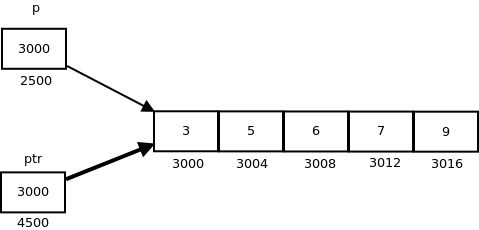# 12.4.1: Pointer to an Array - Array Pointer

$$\newcommand{\vecs}{\overset { \rightharpoonup} {\mathbf{#1}} }$$ $$\newcommand{\vecd}{\overset{-\!-\!\rightharpoonup}{\vphantom{a}\smash {#1}}}$$$$\newcommand{\id}{\mathrm{id}}$$ $$\newcommand{\Span}{\mathrm{span}}$$ $$\newcommand{\kernel}{\mathrm{null}\,}$$ $$\newcommand{\range}{\mathrm{range}\,}$$ $$\newcommand{\RealPart}{\mathrm{Re}}$$ $$\newcommand{\ImaginaryPart}{\mathrm{Im}}$$ $$\newcommand{\Argument}{\mathrm{Arg}}$$ $$\newcommand{\norm}{\| #1 \|}$$ $$\newcommand{\inner}{\langle #1, #2 \rangle}$$ $$\newcommand{\Span}{\mathrm{span}}$$ $$\newcommand{\id}{\mathrm{id}}$$ $$\newcommand{\Span}{\mathrm{span}}$$ $$\newcommand{\kernel}{\mathrm{null}\,}$$ $$\newcommand{\range}{\mathrm{range}\,}$$ $$\newcommand{\RealPart}{\mathrm{Re}}$$ $$\newcommand{\ImaginaryPart}{\mathrm{Im}}$$ $$\newcommand{\Argument}{\mathrm{Arg}}$$ $$\newcommand{\norm}{\| #1 \|}$$ $$\newcommand{\inner}{\langle #1, #2 \rangle}$$ $$\newcommand{\Span}{\mathrm{span}}$$$$\newcommand{\AA}{\unicode[.8,0]{x212B}}$$

Pointer to Array

Consider the following program:

#include <iostream>
using namespace std;

int main()
{
int myArr = { 1, 2, 3, 4, 5 };
int *newPtr = myArr;

cout << "Pointer is " << newPtr << endl;
return 0;
}


In this program, we have a pointer newPtr that points to the 0th element of the array. Similarly, we can also declare a pointer that can point to whole array instead of only one element of the array. This pointer is useful when talking about multidimensional arrays.
Syntax:

data_type (*var_name)[size_of_array];


Example:

int (*ptr);

Here ptr is pointer that can point to an array of 10 integers. Since subscript have higher precedence than indirection, it is necessary to enclose the indirection operator and pointer name inside parentheses. Here the type of ptr is ‘pointer to an array of 10 integers’.

Note : The pointer that points to the 0th element of array and the pointer that points to the whole array are totally different. The following program shows this:

// C++ program to understand difference between
// pointer to an integer and pointer to an
// array of integers.
#include <iostream>
using namespace std;

int main()
{
// Pointer to an integer
int *p;

// Pointer to an array of 5 integers
int (*ptr);
int arr;

// Points to 0th element of the arr.
p = arr;

// Points to the whole array arr.
ptr = &arr;

cout << "p = " << p << ", ptr = " << ptr << endl;
p++;
ptr++;
cout << "p = " << p << ", ptr = " << ptr << endl;

return 0;
}


The following output shows that when the point p get incremented the address only increases by 4 - because an integer is 4 bytes long, and we therefore need to move to the beginning of the next member of the array. The variable ptr points to the entire array, which is 5 integers of 4 bytes each - so the array is 20 bytes long. When we increment the variable ptr it increases by 20 - remember looking at the output that we are dealing with hexadecimal values...

p = 0x7fff4f32fd50, ptr = 0x7fff4f32fd50
p = 0x7fff4f32fd54, ptr = 0x7fff4f32fd64

p: is pointer to 0th element of the array arr, while ptr is a pointer that points to the whole array arr.

• The base type of p is int while base type of ptr is ‘an array of 5 integers’.
• We know that the pointer arithmetic is performed relative to the base size, so if we write ptr++, then the pointer ptr will be shifted forward by 20 bytes.

The following figure shows the pointer p and ptr. Darker arrow denotes pointer to an array.On dereferencing a pointer expression we get a value pointed to by that pointer expression. Pointer to an array points to an array, so on dereferencing it, we should get the array, and the name of array denotes the base address. So whenever a pointer to an array is dereferenced, we get the base address of the array to which it points.

// C++ program to illustrate sizes of
// pointer of array
#include <bits/stdc++.h>
using namespace std;

int main()
{
int arr[] = { 3, 5, 6, 7, 9 };
int *p = arr;
int (*ptr) = &arr;

cout << "p = "<< p <<", ptr = " << ptr << endl;
cout << "*p = "<< *p <<", *ptr = " << *ptr << endl;

cout << "sizeof(p) = " << sizeof(p) <<  ", sizeof(*p) = " << sizeof(*p) << endl;
cout << "sizeof(ptr) = " << sizeof(ptr) << ", sizeof(*ptr) = " << sizeof(*ptr) << endl;

return 0;
}


This is interesting output. The 2 pointers have the same address. The sizeof shows a couple of things: 1) the sizeof for both p and ptr shows 8 bytes - the amount of memory necessary to store an address; 2) the sizeof(*p) is 4, because this pointer points to a single integer value; 3) the sizeof(*ptr) is 20 because it points to the entire array of 5 integer values, or 20 bytes.

p = 0x7ffde1ee5010, ptr = 0x7ffde1ee5010
*p = 3, *ptr = 0x7ffde1ee5010
sizeof(p) = 8, sizeof(*p) = 4
sizeof(ptr) = 8, sizeof(*ptr) = 20


This page titled 12.4.1: Pointer to an Array - Array Pointer is shared under a CC BY-SA license and was authored, remixed, and/or curated by Patrick McClanahan.